You’re using an older browser version. Update to the latest version of Google Chrome, Safari, Mozilla Firefox, or Microsoft Edge for the best site experience.

# Calculation of a passing score in SCORM

PRODUCTS:   Suite,   QuizMaker,   Presenter

Usually, a learning course is a combination of slides and quizzes. For example, this learning course contains 50 slides and two of them are occupied by iSpring quizzes: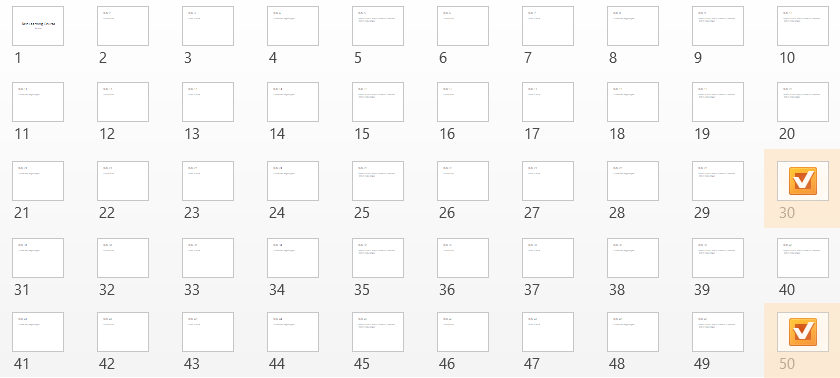You can choose how slides and quizzes are rated by configuring the Report Progress and Completion settings before publishing a presentation. Each item with a Weight assigned to it represents a learning activity that affects the calculation of a learner’s score by an LMS, which will ultimately decide whether the course is passed or failed.

iSpring Publish > LMS > Learning Course tab > "Customize..." button: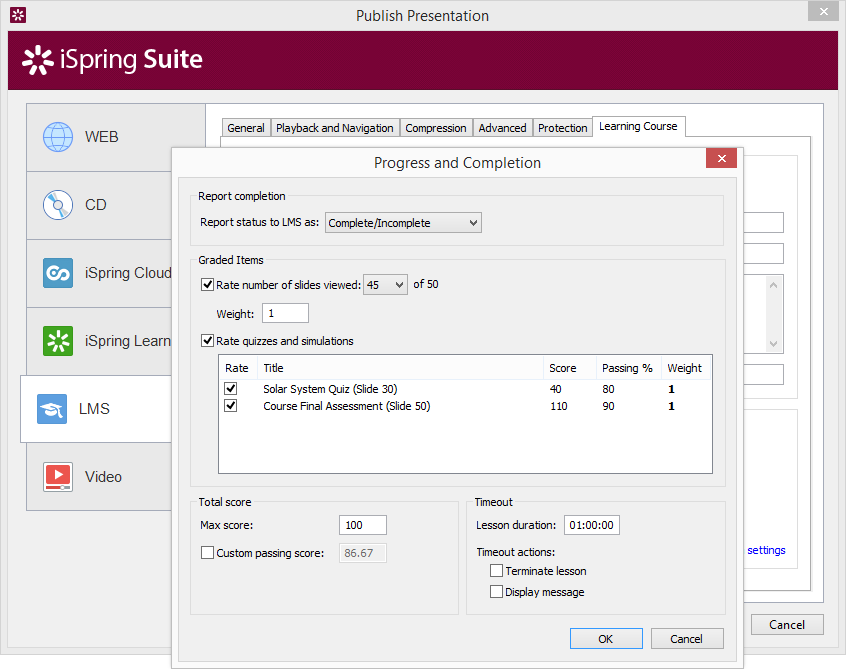On this screenshot above, the full course's score consists of 3 parts with their local passing scores. Each part contributes 1/3 to the overall passing score.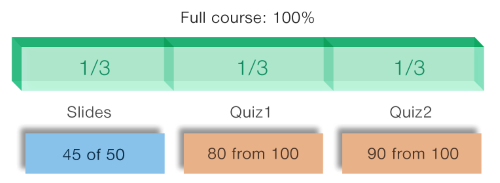If a learner views 50 slides, and gets 100% score for both quizzes, the overall score will be 100%. If the learner completes 45 slides, gains 80% for the Quiz1 and 90% for the Quiz2, the score will be 86.7% which is a passing score.

On the settings picture above at the very bottom where it says Custom passing score you can see the grayed out number. But how did iSpring figure out that the optimum passing score for the entire course is 86.7?

The Passing Score is calculated automatically based on the Max Score and other parameters of the learning activities. This procedure applies equally to both SCORM 1.2 and 2004 courses. Let's take a look behind the curtains and see how this automatic calculation works.

Passing score is a weighted arithmetic mean of all passing scores in the course.

Simplified equation: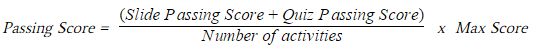An iSpring course structure features 2 activity types used for grading:

• Slide Passing Score. It is a percent value of slides, necessary for viewing from all slides.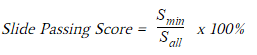• Quiz Passing Score. A course may include one or several quizzes. If we have more than one quiz, the final quiz passing score will be equal to the sum of their passing scores: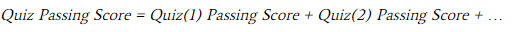The number of activities is equal to 1 when we rate slide progress only (or a single quiz without slides). It becomes 2 if we rate slide progress and 1 quiz. It is 3 when we have 2 quizzes plus slides, etc.

Max Score is usually 100, unless you grade your learners using a different scale than percentage.

### Example 1:

A course consists of 50 slides and doesn’t have any quizzes.

Max score is 100.

Number of slides necessary to view is 40.

Here is the formula for the final passing score: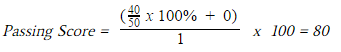iSpring Suite tab in PowerPoint. Publish > LMS > Learning Course tab: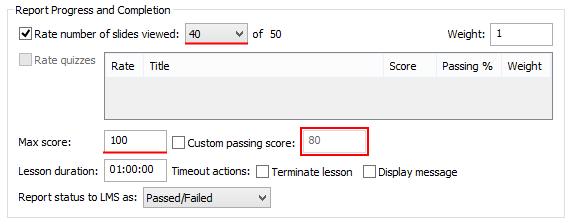### Example 2:

A course consists of 50 slides and 1 quiz (added on the last slide).

Max score is 100.

Number of slides necessary to view is 40 (This number includes the slide that contains the quiz).

Quiz passing score is 90%.

Here is the formula for the final passing score: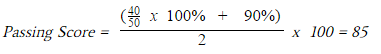iSpring Suite tab in PowerPoint. Publish > LMS >Learning Course tab: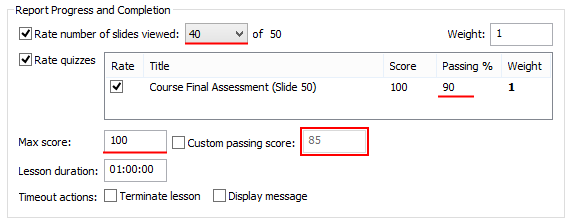iSpring QuizMaker (as a part of Suite). Quiz Properties > Main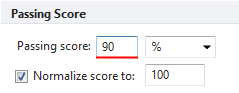## Assigning weights to learning activities

Also you can set weights to slide progress and to each individual quiz. Usually, the weight is 1 for each activity. If you set a weight other than 1, it makes the calculation process a bit more complex.

Full equation: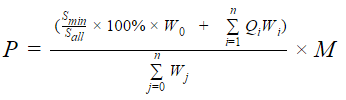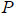– Passing score;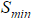– Minimum number of slides a learner has to view;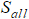– Overall number of slides in the course;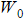– Weight of the slide progress;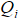– Passing score of a quiz i in %;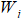– Weight of a quiz i;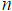– Number of graded quizzes in a course;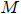– Maximum score;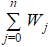– Overall sum of weights for slide progress and all quizzes.

### Example 3:

A course consists of 50 slides and 1 quiz.

Max score is 100.

Number of slides necessary to view is 40.

Quiz passing score is 90%.

Quiz Weight is 3.

In this example, we’re considering the assessment part to be more important than slide views. Therefore, we have set a higher weight to the quiz. The denominator doesn’t show the number of activities like in the simplified equation, but the sum of their weights is 1 + 3.

Here is the formula for the final passing score: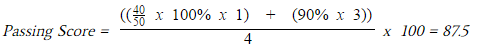iSpring Suite tab in PowerPoint. Publish > LMS > Learning Course tab > Customize button: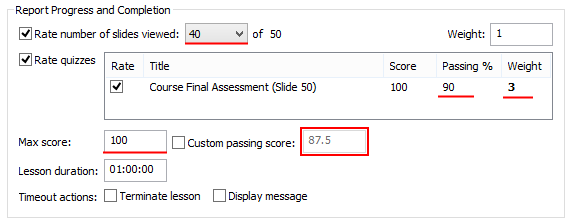At any time you can check the checkbox Custom passing score and set your own value at the publishing step. However, if you don’t bother about course completion, we recommend that you uncheck Rate number of slides viewed and leave just the Rate quizzes option checked.

## FAQ

Q: I have a pool of questions that I want to show to a learner throughout the course. Can I have one quiz that is placed on several slides and still have one reporting score?

A: No you can’t. An iSpring Quiz is a solid object that can be added onto a slide of your PowerPoint presentation but can’t be divided among several questions. However, you can add as many quizzes as you want.

Q: My learners can never reach 100% completion of the course though they viewed all slides and answered to all questions correctly. I rate both: slides and quizzes. What’s wrong?

A: Your presentation might have hidden slides or custom slide shows that can’t be visited by a learner. Please decrease the number of slides necessary to view to the number of visible slides, or don’t rate the number of slides viewed at all. It will resolve this problem.

Q: Can I set weights to different slides in my course?

A: No, you can’t set different weights to selected slides in your presentation. Slide viewing is a single learning activity and it’s graded as a whole.

Q: Is there a limit to the number of quizzes that I can have in my course?

A: Virtually, there is no limitation applied to the number of quizzes added to your course. We performed stress testing and added about 250 quizzes, which didn’t evoke the slightest problem.

Related Articles

### iSpring Suite 11.3

Fully-stocked eLearning authoring toolkit for PowerPoint. No training required to start!